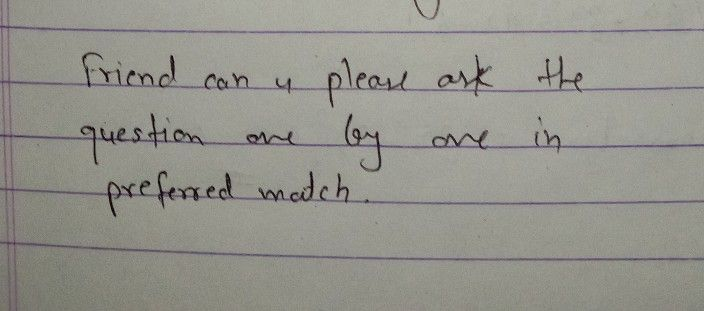Symbol
ProblemActivity 2: Lat's Write an Equation! $e→cns$ Write $-$ equation of the line in $clop0$ intercept form given the $010\times 9$ 1. The line passes the points $-6$ $21$ $and\left(3-5\right)$ $2$ The line passes the point $\left(3-4\right)an$ a slope of 3. $3.$ $A$ line that has a slope $g$ $2anda$ $y-ntcrccpt$ $0$ 4. The line passes through the points $\left(-1.3\right)and\left(2.0\right)$ 5. The line has a slope $20$ -3 and passes through the point $\left(2.11$
7th-9th grade
Other
Search count: 105
Question content
teacher I need a help
SolutionQanda teacher - Aayushiplzzz??Student
ok 1. the line passes the point (-6, 2) and (3, -5)
plss teacher